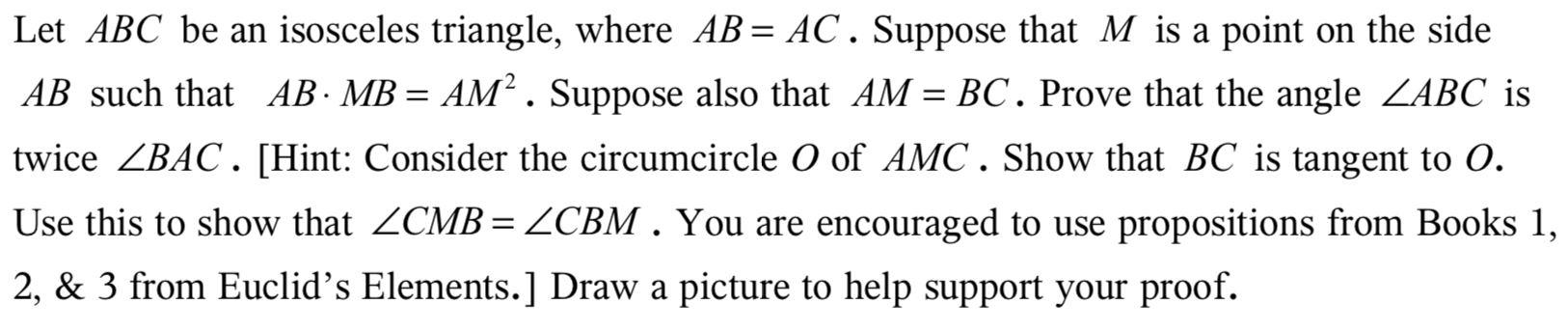Let ABC be an isosceles triangle, where AB AC. Suppose that M is a pointon the sideAB such that AB- MB = AM2. Suppose also that AM = BC. Prove that the angle ZABC istwice ZBAC. [Hint: Consider the circumcircle O of AMC. Show that BC is tangent to O.Use this to show that ZCMB = ZCBM . You are encouraged to usepropositions from Books 1,2, & 3 from Euclid's Elements.] Draw a picture to help support your proof.

Questionhelp_outlineImage TranscriptioncloseLet ABC be an isosceles triangle, where AB AC. Suppose that M is a point on the side AB such that AB- MB = AM2. Suppose also that AM = BC. Prove that the angle ZABC is twice ZBAC. [Hint: Consider the circumcircle O of AMC. Show that BC is tangent to O. Use this to show that ZCMB = ZCBM . You are encouraged to use propositions from Books 1, 2, & 3 from Euclid's Elements.] Draw a picture to help support your proof. fullscreen
Step 1

Consider the isosceles triangle having sides AB,BC and AC such as AB=AC.On side AB there is a point M such that AM=BC and .Also triangle AMC have a circumcircle O.Write the given data about this triangle:

Step 2

Now draw the triangle based on above information.

Step 3

Condition for tangency of a line can be ex...

Want to see the full answer?

See Solution

Want to see this answer and more?

Our solutions are written by experts, many with advanced degrees, and available 24/7

See Solution
Tagged in

Triangles